Specifying the Margin of Error

Instead of specifying the total sample size to allocate among the strata, you can specify the desired margin of error for estimating the overall mean from the stratified sample. Based on the requested allocation method and the stratum variances that you provide, PROC SURVEYSELECT computes the stratum sample sizes that are required to achieve this margin of error. You specify the margin of error in the MARGIN= option in the STRATA statement, and you provide stratum variances in the VAR= option. You can use the MARGIN= option with any allocation method (proportional, optimal, or Neyman) or with allocation proportions that you provide (ALLOC=(values) or ALLOC=SAS-data-set).

The margin of erroris the half-width of the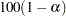% confidence interval for the overall mean based on the stratified sample,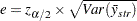where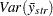is the variance of the estimate of the mean from the stratified sample and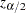is the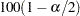th percentile of the standard normal distribution. You can specify the value ofin the ALPHA= option in the STRATA statement. By default, PROC SURVEYSELECT uses a 95% confidence interval (ALPHA=0.05).

For the specified margin of error, PROC SURVEYSELECT computes the target stratum sample sizes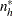for without-replacement selection methods as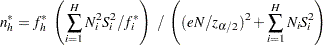where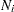is the number of sampling units in stratum,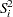is the variance within stratum,
is the total number of sampling units for all strata, and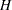is the total number of strata.

The values of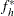are the stratum allocation proportions, which PROC SURVEYSELECT computes according to the allocation method that you request. See the sections Proportional Allocation, Optimal Allocation, and Neyman Allocation for details.

For with-replacement selection methods, PROC SURVEYSELECT computes the target stratum sample sizes as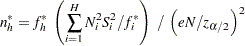See Lohr (2010, page 91), Cochran (1977, Chapter 5), and Arkin (1984, Chapter 10) for more information.

The target sample size valuesmight not be integers, but the stratum sample sizes are required to be integers. PROC SURVEYSELECT rounds all fractional target sample sizes up to integer sample sizes. If you specify a minimum stratum sample size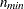in the ALLOCMIN= option in the STRATA statement, then all stratum sample sizes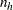are required to be at least.

For without-replacement selection methods, a stratum sample size cannot exceed the number of units in the stratum. If a target stratum sample size does exceed the number of units in the stratum, the procedure sets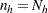for that stratum, removes the stratum from the variance computation (because it contributes nothing to the sampling error), revises the allocation proportionsfor the remaining strata, and computes the stratum sample sizes again. If a stratum sample size equals the number of units in its stratum, the procedure also removes that stratum from the variance computation and revises the sample sizes for the remaining strata. See Cochran (1977, page 104) and Arkin (1984, page 176) for details.

When you specify the STATS option with the MARGIN= option in the STRATA statement, PROC SURVEYSELECT displays the expected margin of error for the sample allocation. The expected margin of error (for the overall mean based on the stratified sample) is computed from the stratum sizes (), the stratum variances that you provide (), and the allocated stratum sample sizes that the procedure computes (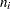). For without-replacement selection methods, the expected margin of error is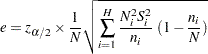For with-replacement selection methods, the expected margin of error is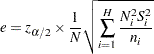The expected margin of error should be less than or equal to the value specified in the MARGIN= option. Any difference between the expected margin and the specified value is due to rounding the target stratum sample sizes up to integer values and increasing stratum sample sizes to equal the required minimum value (ALLOCMIN=).